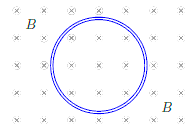# Problem: A single circular loop of wire in the plane of the page is perpendicular to a uniform magnetic field ~B directed into the page, as shown. If the magnitude of the magnetic field is decreasing, then the induced current in the wire loop is 1. counterclockwise around the loop. 2. directed upward out of the paper. 3. zero. (No current is induced.) 4. clockwise around the loop. 5. directed downward into the paper.

🤓 Based on our data, we think this question is relevant for Professor Sinkovic's class at UCONN.

###### Problem Details

A single circular loop of wire in the plane of the page is perpendicular to a uniform magnetic field ~B directed into the page, as shown. If the magnitude of the magnetic field is decreasing, then the induced current in the wire loop is

1. counterclockwise around the loop.

2. directed upward out of the paper.

3. zero. (No current is induced.)

4. clockwise around the loop.

5. directed downward into the paper.